# Martingale strategies don’t work, but we knew that – Simulation analysis in R

April 5, 2019
By

Want to share your content on R-bloggers? click here if you have a blog, or here if you don't.It’s generally accepted that Martingale betting strategies don’t work. But people still gravitate towards them because they are intuitive. I was curious to find out how they actually perform.

Disclaimer: I do not encourage gambling, nor do I gamble myself but the games are good examples of stochastic processes.

## At a glance

• The classic and Reverse Martingale strategies don’t work. They will almost certainly have you leaving with less money than what you started with, or more often than not no money at all.
• The reverse strategy is generally less risky, higher potential to win big but lower returns on average.
• Going all in on the first bet is the best way to double your money and save your time.

## Classic Martingale

The Classic Martingale strategy is as follows. Make an initial bet $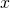. If you win, bet$on the next round. If you lose, double your previous bet. In a nutshell you bet $where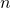is the number of losses in a row. The intention is to make your money back on the next win. Assume we start with$100 and our initial bet is $1. We keep playing until there is not enough money in the cash pool to make the next bet. Also assume we are playing roulette on an American table and place bets on red or black which both have the probability 18/38. It only takes a streak of 6 losses before the game is over because we don’t have enough cash to double our bet for a 7th time. If we see a streak of 10 or more it really starts to get out of control. trials bet cumulative_loss 1 1 1 2 2 3 3 4 7 4 8 15 5 16 31 6 32 63 7 64 127 8 128 255 9 256 511 10 512 1023 The probability of losing 6 in a row is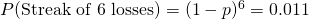. Sounds unlikely, but it will occur more often than you think. With each win we will win$1, so once we have won 27 times we’ll have enough cash in order to afford a losing streak of 6 and bet on the 7th.

It’s more likely we’ll have a few wins and losses before observing a long losing streak that takes us out of the game. The question is how many trials (spins of the roulette wheel) will we place bets on before we lose our money and play stops? A slight variation I’ve applied is, if there is not enough money left to double the bet, we will simply bet the remainder in the cash pool, in other words go all-in. More in line with what someone might do.

This simulation was a relatively lucky one, winning over $170 and almost 400 trials, however one bad streak and it’s all over. You can plot as many simulations as you like, some are shorter and some are longer but they all end the same way.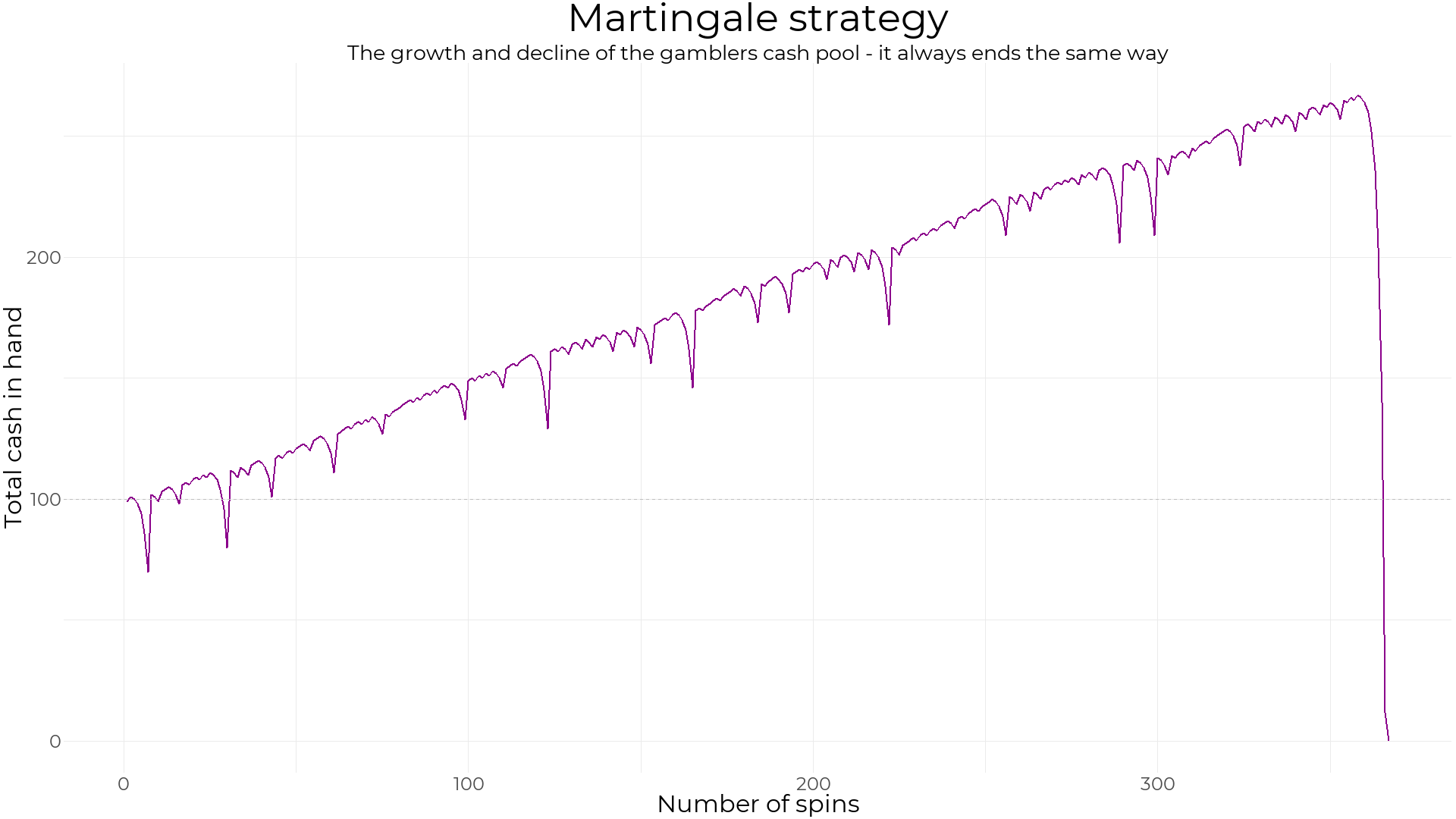This is a typical pattern for the classic strategy. If you wish to see more try running the code at the end of this post or view this set. ## Reverse Martingale Instead of doubling the bet upon a loss, double the bet upon a win. Not surprisingly this ends the same way as the classic strategy. The players cash amount slowly decreases by$1 on each loss. Occasionally there is a big win, rather than a big loss. With this strategy you can watch your money dwindle away rather than vanish in front of your eyes.

This plot is using the same win-lose sequence as the one above. In this case the maximum cash held throughout the game is higher with the classic strategy than the reverse.Click here to see more simulations.

## Number of trials for each strategy

These strategies was simulated 20,000 times. The distribution of the number of trials shows how long a typical game will last until bankruptcy. The classic strategy has a very long tail, so potentially could be playing for a very long time. The maximum number of trials is this simulation was 64254. But you could also be playing for a very short time.

The reverse strategy has a slightly higher median number of trials but much less variable than the classic strategy meaning you can be sure to play between 166 and 217 spins.min 2.5% 10% 50% 90% 97.5% max
classic 7 10 22 170 1132 2759 64254
reverse 152 167 172 191 207 213 226

Assume the goal is to double your money. What is the probability you’ll double your money before going bust? After 20,000 simulations for both strategies, the probability you will double your money using…

• The Classic Martingale strategy is 0.35
• The Reverse Martingale strategy is 0.28

The Classic Martingale strategy tends to do better on average, but only slightly. Neither of these strategies are better than simply playing once and going all-in which is 0.47

## Maximum cash

The distribution of the maximum amount of cash held by the player at any given time during the game shows the classic strategy has the slight edge over the reverse strategy on average. Although the reverse strategy has the potential for massive wins if you score a good winning streak.min 2.5% 10% 50% 90% 97.5% max
classic 100 101 107 158 425 926 19894
reverse 100 100 102 133 534 2062 131163However, keep in mind these simulations all resulted in total loss at the end of the game. Perhaps the key is to know when to stop?

## Stopping conditions

Rather than stopping once you reach a specified amount which may not happen, stop when the bet reaches a specified amount.

We could specify a streak length, however a better idea is to specify a ratio of bet to total cash. This way the is stopping condition is dynamic. For example, if there is a winning streak we’ll have more money in which to bet.

Essentially by using this ratio we are fixing a certain level risk rather than betting amount. The ratio is calculated asIf we fix a stopping ratio of 0.1, we could place 4 bets before the ratio exceeds 0.1. If our initial cash pool was \$200 we could place 5 bets until the ratio exceeds 0.1.

If we stop as soon as this ratio is reached it means we’re on a losing streak so it makes sense in the case of the classic strategy to bet again until our next win and walk away.

In the case of the reverse it makes sense to leave as soon as the ratio is met since we’re on a winning streak.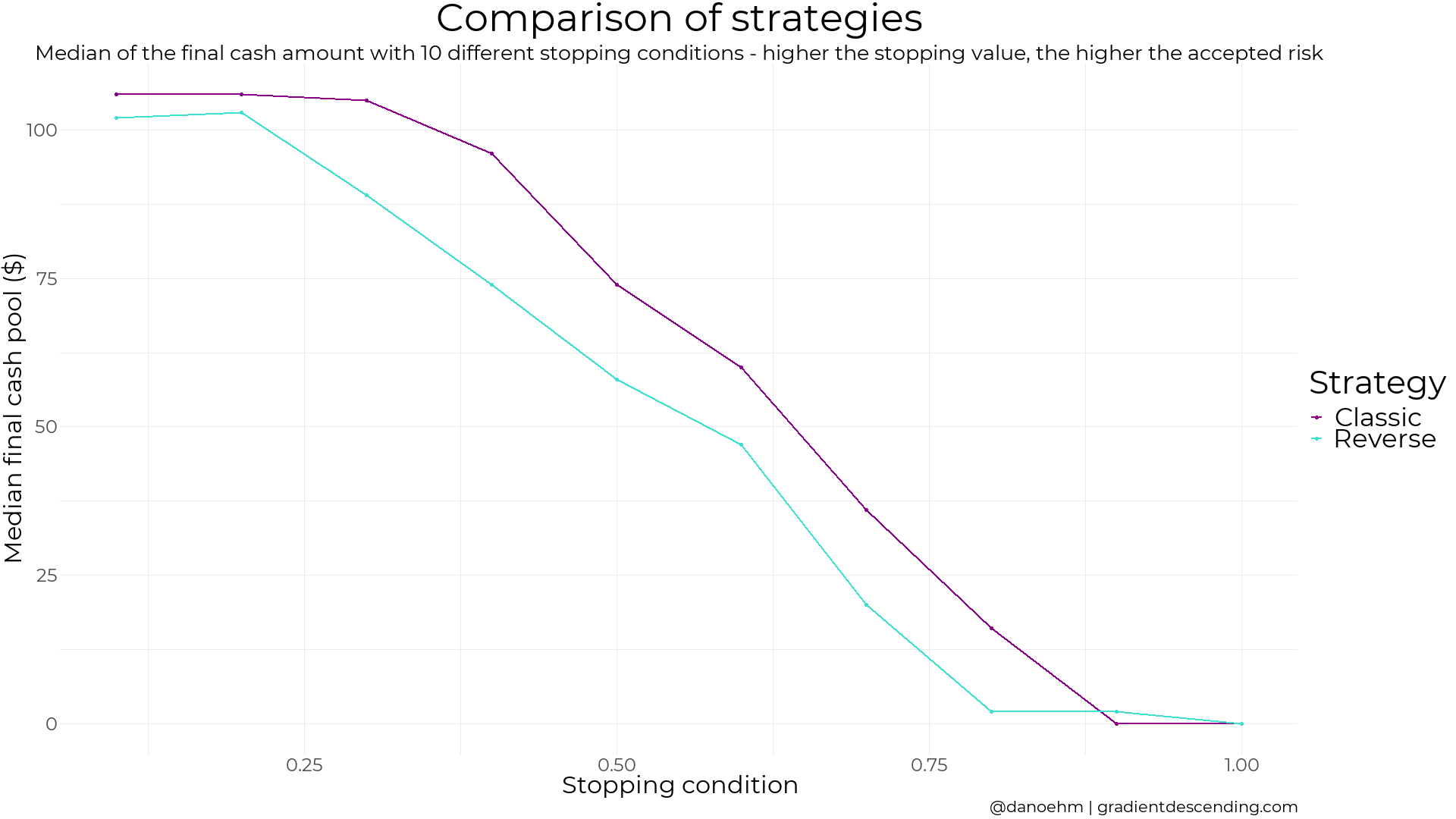There are clear differences and similarities between the two strategies.

• The median final amount for the low risk stopping condition is actually slightly above 100 for both strategies, meaning it is likely you will break even or take home a couple of bucks.
• As the risk goes up the median value decreases where the classic strategy tends to have a higher median than the reverse strategy.
• Higher the risk, the higher potential for bigger wins.
• The reverse strategy has some interesting features. The ‘bumps’ occur when the risk is great enough to allow the next bet when you’re on a streak. However the chance of this streak is low enough that it doesn’t improve the strategy.

Overall the stopping strategies help to minimise loss rather than lock in wins, so on average you will still lose your money. The Martingales don’t work.

## Code bits

The code for the plots in this post can be found on github. The Martingale function is below.

# libraries
library(tidyverse)

# martingale function
martingale <- function(bet, cash, p, stop_condn = Inf, stop_factor = Inf, reverse = FALSE, plot = TRUE, stop_on_next_win = TRUE){

bet_vec <- vector(mode = "numeric")
cash_vec <- vector(mode = "numeric")
outcome <- vector(mode = "numeric")
winnings <- vector(mode = "numeric")
total_cash <- vector(mode = "numeric")
trial <- 0
total_cash <- cash

while(total_cash[max(trial, 1)] > 0){

# iterate through trials
trial <- trial + 1

# update cash pool
if(trial == 1){
cash_vec[trial] <- cash
}else{
cash_vec[trial] <- total_cash[trial-1]
}

# set bet
if(!reverse){
if(outcome[trial - 1] == 1 || trial == 1){
bet_vec[trial] <- bet
}else{
bet_vec[trial] <- min(2*bet_vec[trial-1], cash_vec[trial]) # if there isn't enough to double the bet just bet what is left
}
}else{
if(outcome[trial - 1] == 0 || trial == 1){
bet_vec[trial] <- bet
}else{
bet_vec[trial] <- min(2*bet_vec[trial-1], cash_vec[trial]) # if there isn't enough to double the bet just bet what is left
}
}

# stop condition
if(bet_vec[trial]/cash_vec[trial] > stop_condn){      if(stop_on_next_win & !reverse){        stop_trigger <- TRUE      }else{        outcome[trial] <- NA        winnings[trial] <- NA        total_cash[trial] <- cash_vec[trial]        break      }    }

outcome[trial] <- sample(c(0,1), 1, prob = c(1-p, p))
winnings[trial] <- bet_vec[trial]*outcome[trial] - bet_vec[trial]*(1-outcome[trial])
total_cash[trial] <- cash_vec[trial] + winnings[trial]

# stop condition
if(total_cash[trial] >= stop_factor*cash) break
}

# make the plot
g1 <- NULL
if(plot){
df <- data.frame(trials = 1:trial, cash = total_cash)
gg <- ggplot() +
geom_line(data = df, mapping = aes(x = trials, y = cash), col = "darkmagenta", lty = 1, size = 1) +
geom_hline(yintercept = cash_vec, col = "grey", lty = 2) +
theme_minimal() +
labs(
x = "Number of spins",
y = "Total cash in hand",
title = ifelse(reverse, "Reverse Martingale strategy", "Martingale strategy"),
subtitle = "The growth and decline of the gamblers cash pool - it always ends the same way"
) +
ylim(0, NA)
print(gg)
}

return(list(
bet = bet_vec,
cash = cash_vec,
outcome = outcome,
total_cash = total_cash,
trials = trial,
plot = gg))
}

# run the simulation and plot the output
# try different parameters to see the effect
martingale(1, 100, 18/38, reverse = FALSE, plot = TRUE, stop_condn = 1,    stop_on_next_win = TRUE)

R-bloggers.com offers daily e-mail updates about R news and tutorials about learning R and many other topics. Click here if you're looking to post or find an R/data-science job.
Want to share your content on R-bloggers? click here if you have a blog, or here if you don't.